Reconstruction of the 2014 Nettle Hill formation 1.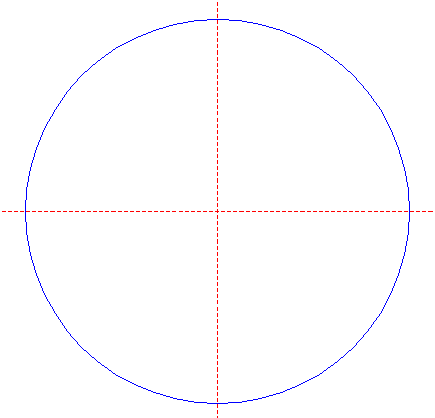Draw a circle. Draw and extend the horizontal and vertical centerlines. 2.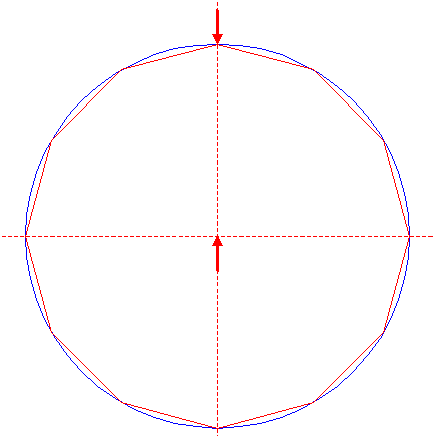Construct the inscribed dodecagon (regular 12-sided polygon) of circle 1, pointing up. 3.Construct the inscribed circle of dodecagon 2. 4.Construct the inscribed regular 24-sided polygon of circle 3, pointing up. Number the angular points 1 - 24, counterclockwise, starting at the top. 5.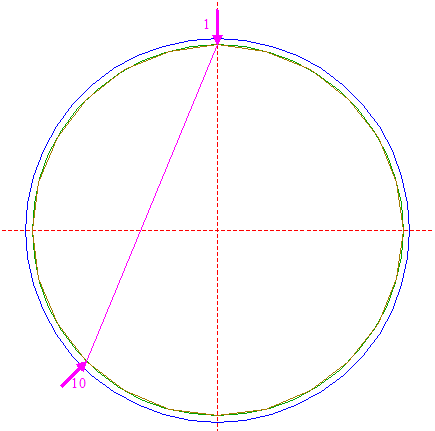Draw the diagonal of polygon 4, between angular points nrs. 1 and 10 (diagonal 1-10). 6.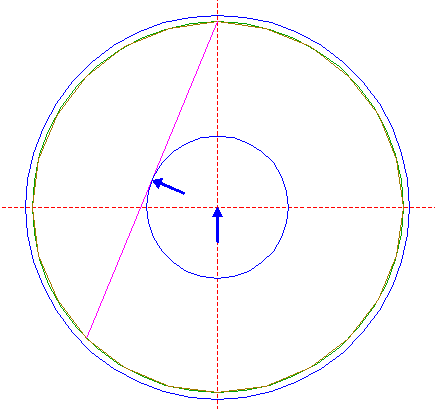Construct a circle concentric to circle 1, tangent to line 5. 7.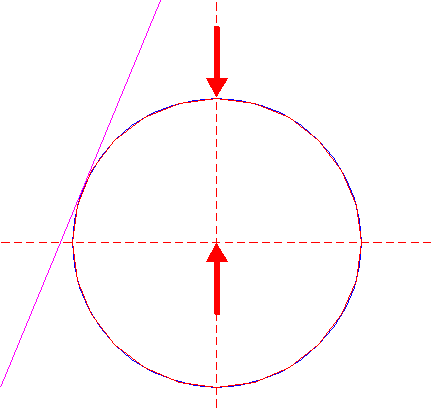Construct the inscribed regular 24-sided polygon of circle 6, pointing up. 8.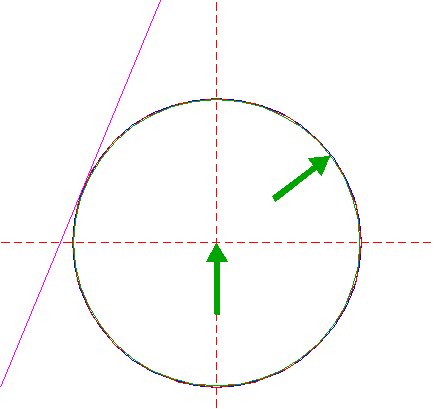Construct the inscribed circle of polygon 7. 9.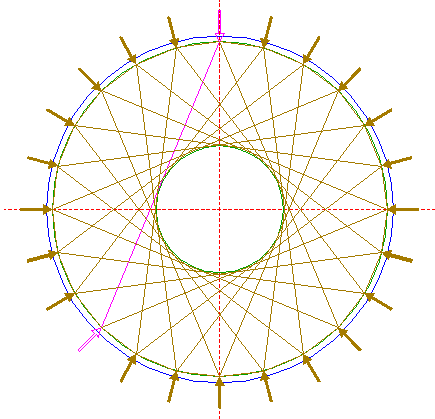Repeat step 5 twenty-three times, relative to all other angular points of polygon 4, as shown. 10.Construct a circle concentric to circle 1, passing through the intersection of line 5 and the horizontal centerline. 11.Point A is the intersection of line 5 and diagonal 9 6-21. Construct a circle concentric to circle 1, passing through A. See detail.12.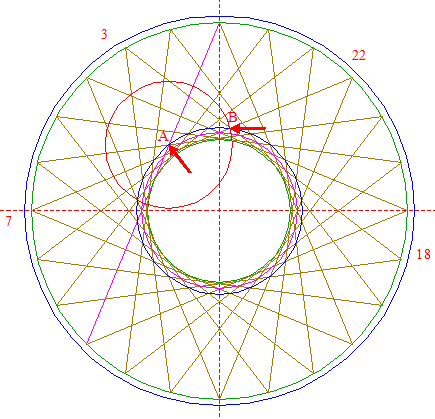Point B is the intersection of diagonals 9 3-18 and 7-22. Construct a circle centered at A, passing through B. See detail.13.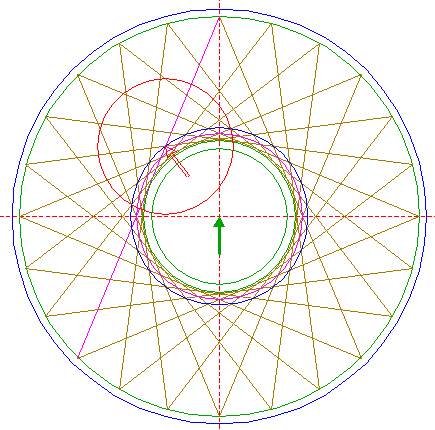Copy circle 12 to the center of circle 1. 14.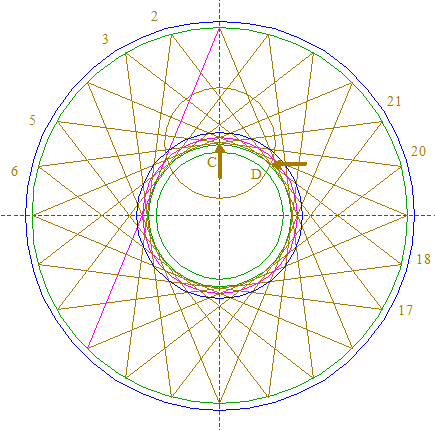Point C is the intersection of diagonals 9 5-20 and 6-21, and point D is the intersection of diagonals 9 2-17 and 3-18. Construct a circle centered at C, passing through D. See detail.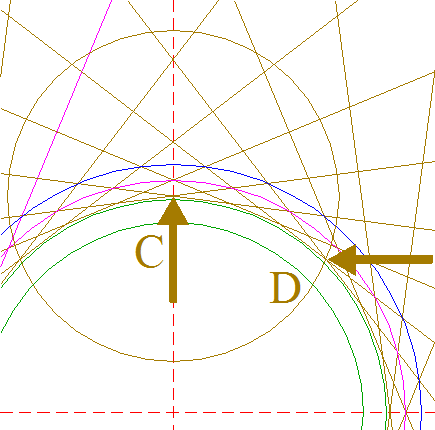15.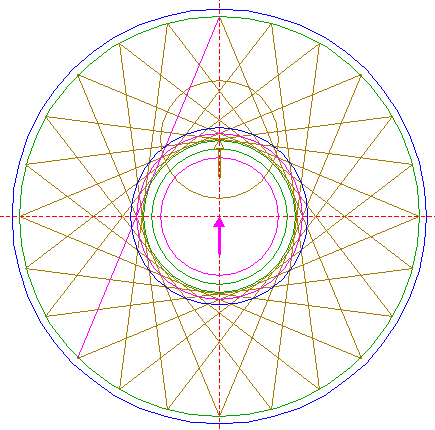Copy circle 14 to the center of circle 1. 16.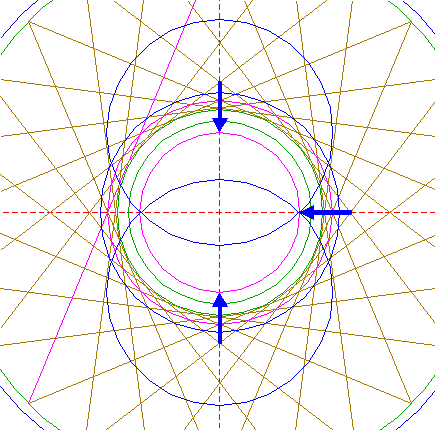Construct two circles, centered at the upper and lower intersections of circle 15 and the vertical centerline respectively, both passing through the righthand intersection of circle 15 and the horizontal centerline. 17.For clarity reasons, some of the results of previous steps are removed temporarily. Draw the connecting line between the intersections of circle 8 and upper circle 16. 18.Construct a circle concentric to circle 1, tangent to line 17. 19.Construct the inscribed regular 24-sided polygon of circle 15, pointing up. Number the angular points similar to polygon 4. 20.Draw the twenty-four rays of polygon 19. 21.Draw seven lines, between angular points 19 nrs. 1 and 7 (1-7), 2-8, 3-9, 4-10, 5-11, 6-12, and 7-13. 22.Copy lines 21, while mirroring with respect to the vertical centerline. 23.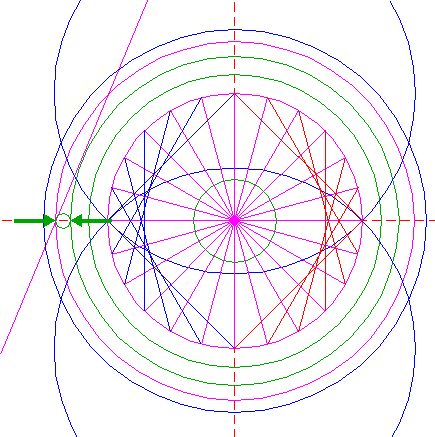Construct a "two-points" circle (defined by the two end-points of a centerline) between the lefthand intersections of circles 8 and 10 with the horizontal centerline. 24.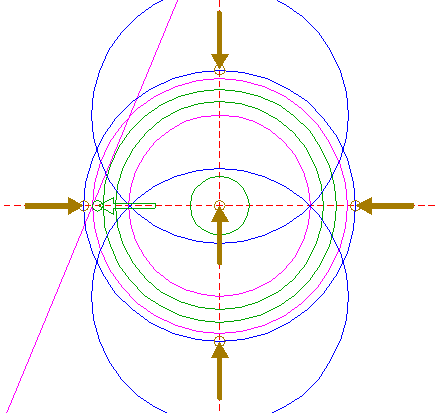Copy circle 23 five times, to the center of circle 1, and to the four intersections of circle 11 with the horizontal and vertical centerlines. 25.Construct two pairs of parallel lines, one horizontal, one vertical, tangent to circles 24 at both sides. 26.Copy circle 23 four times, to the four intersections of central circle 24 with the horizontal and vertical centerlines, and move each of these circles (copy and delete original) to their own corresponding intersection, away from the center of circle 1, as shown. See detail.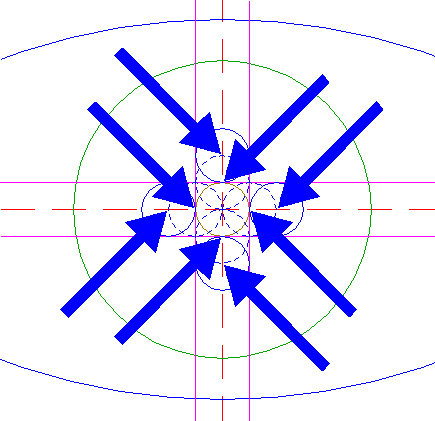27.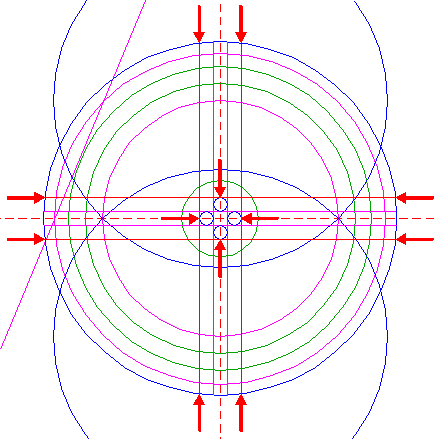Construct two pairs of parallel lines, one horizontal, one vertical, tangent to circles 26 at the outer sides, extending upto circle 11 in both directions, as shown. 28.Construct a circle concentric to circle 1, passing through the intersection of lower circle 16 and ray 20 (to angular point 19) nr. 22. 29.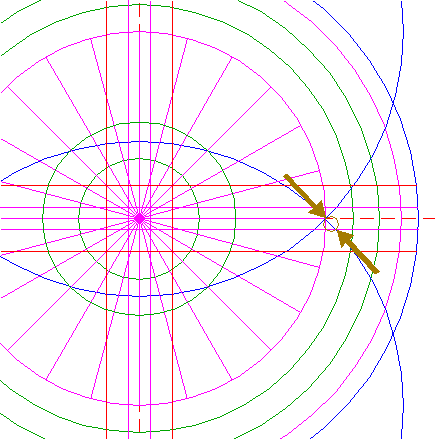Construct a "two-points" circle between the righthand intersections of the horizontal centerline and the lower horizontal line 25 with lower circle 16. 30.Copy circle 29 to the righthand intersection of circle 28 and the horizontal centerline, and move this circle to its own lower intersection with circle 28. 31.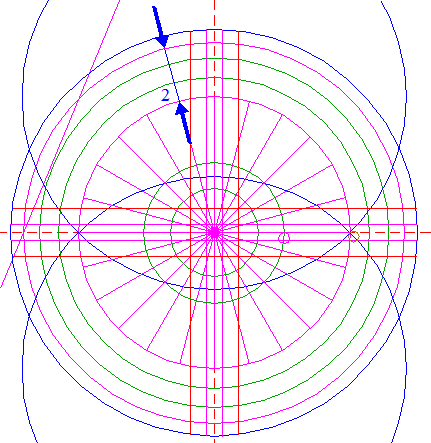Extend ray 20 nr. 2 upto circle 10. 32.Construct a "two-points" circle between the intersections of circles 8 and 10 with line 31. 33.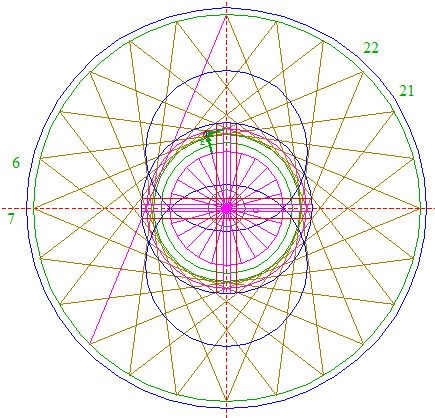Point E is the intersection of diagonals 9 6-21 and 7-22. Construct a circle concentric to circle 32, passing through E. See detail.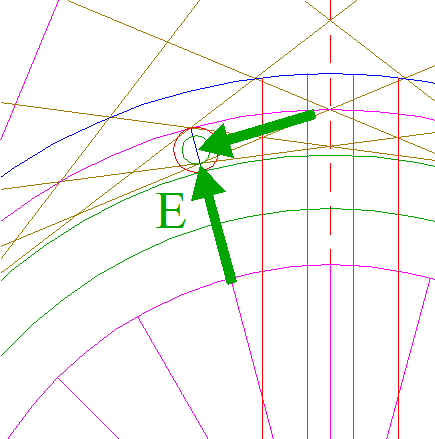34.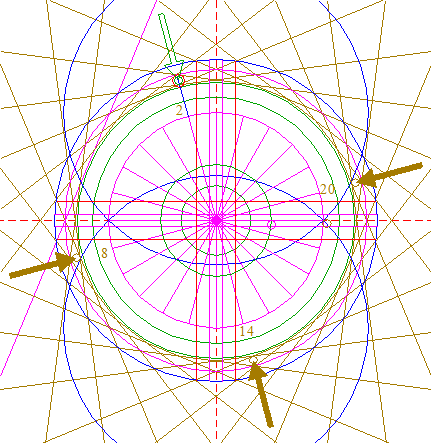Copy circle 33 three times, to the corresponding positions relative to rays 20 nrs. 8, 14 and 20. 35.Construct a circle centered at the intersection of diagonal 9 5-14 and the vertical centerline, tangent to circle 8 at the lower side. See detail.36.Copy circle 35 to the lower intersection of circle 1 and the vertical centerline. 37.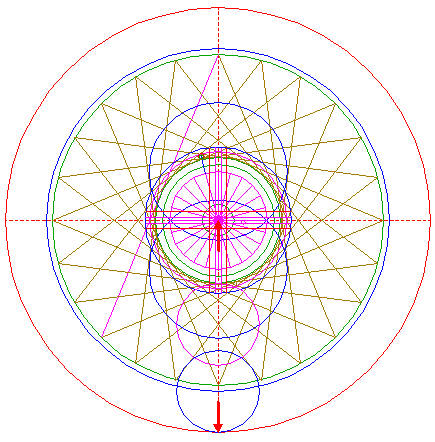Construct a circle concentric to circle 1, tangent to circle 36 at the lower side. 38.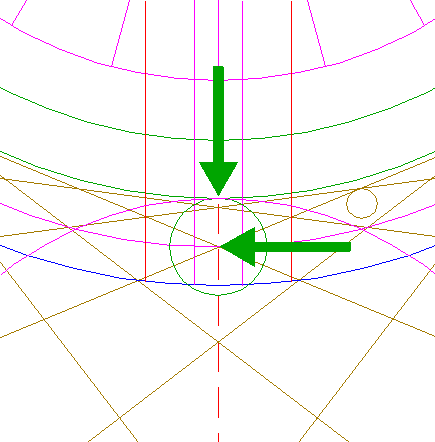Construct a circle centered at the lower intersection of circle 10 and the vertical centerline, tangent to circle 8 at the lower side. 39.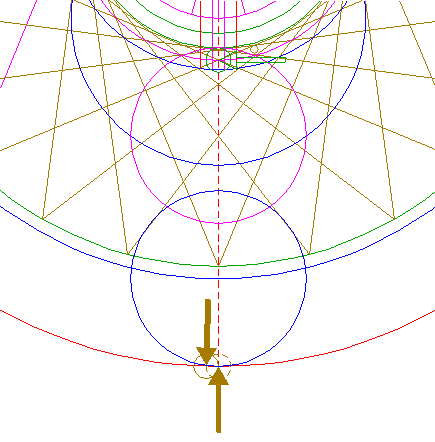Copy circle 38 to the lower intersection of circle 37 and the vertical centerline, and move this circle to its own lefthand intersection with circle 37. 40.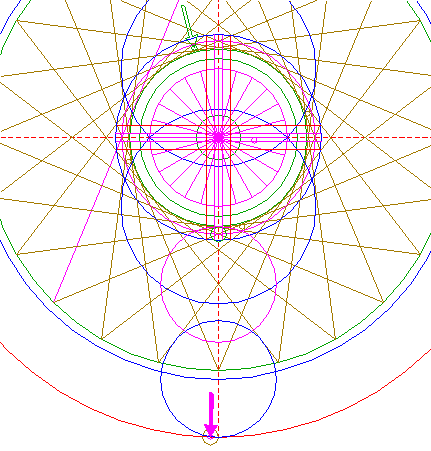Copy circle 33 to the center of circle 39. See detail.41.Circles 1, 3, 8, 10, 11, 13, 15, 16, 18, 30, 33, 34, 39 and 40, and lines 5, 9, 20, 21, 22, 25 and 27, are used for the final reconstruction. 42.Remove all parts not visible within the formation itself. 43.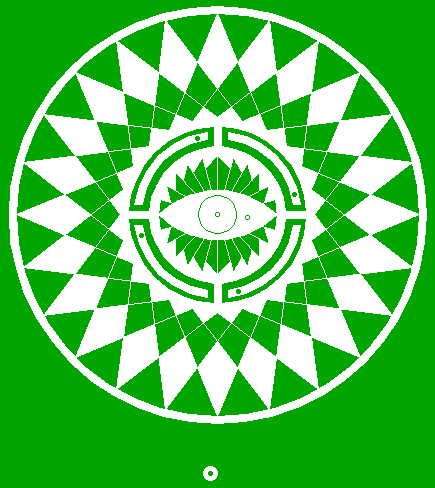Colour all areas corresponding to standing... 44.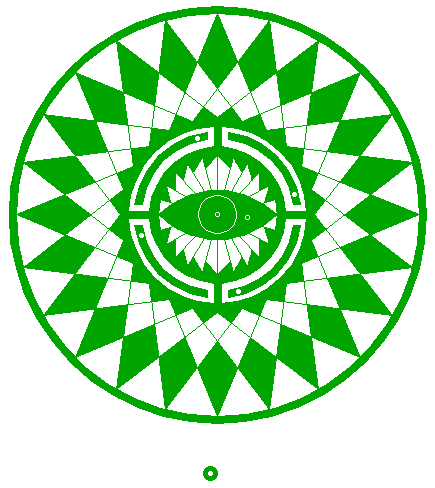...or to flattened crop, and finish the reconstruction of the 2014 Nettle Hill formation. 45.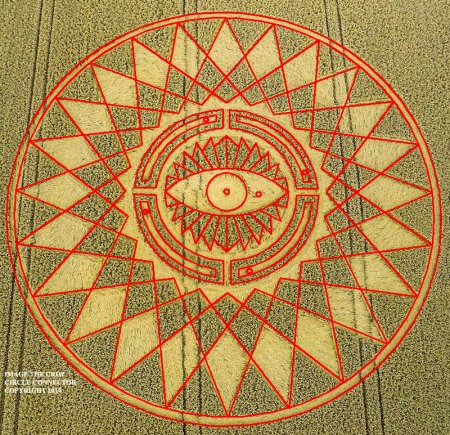The final result, matched with two aerial images.
 Copyright © 2014, Zef Damen, The NetherlandsBACKMark Fussell & Stuart Dike